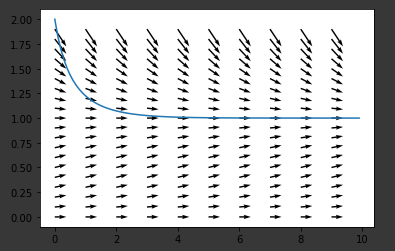# Let's look at a differential equation that cannot be solved normally in Python

## Euler method

When $\ dot {x} = f (x)$, I would like to find a function $g$ that ideally integrates $x$ over time so that $x = g (t)$. However, analytical integration is not possible in nonlinear systems. Therefore, let us consider solving it numerically by discretizing the time. It would be good if we could find a numerical value similar to the integral of $f (x)$ over time for each t. Therefore, the Euler method, which is an application of the segmented quadrature method that combines rectangles, can be used.

## Runge-Kutta method

The Euler method adopted one end (for the younger one) and the modified Euler method adopted both ends as the height of a rectangle, but the Runge-Kutta method is a more elaborate selection of the numerical values ​​to be adopted. The runge-Kutta method of the fourth order is just right in terms of the balance with the amount of calculation. In the quaternary Runge-Kutta method, the rectangular values ​​are calculated for the four points in the interval, and the integrated values ​​in the interval are calculated by averaging with weights. Specifically as follows

x_{n+1}=x_n+\frac{1}{6}(k_1+2k_2+2k_3+k_4)
k_1 = f(x_n)\Delta t
k_2 = f(x_n+\frac{1}{2}k_1)\Delta t
k_3 = f(x_n+\frac{1}{2}k_2\Delta t
k_4 = f(x_n+\frac{1}{2}k_3)\Delta t

Often used is Runge-Kutta to draw the solution trajectory when given the most interesting initial value many times like a graph of a function, and give various other initial values ​​to Runge- You can grasp the whole atmosphere by trying Kutta only once for each and drawing it as a vector field or a tilt field. I will implement it below.

import numpy as np
import matplotlib.pyplot as plt

#Define the function you want to look up
def dotx(x):
return x*(1-x)

#Runge-Kutta method-Plot on x plane
def rk4th(x0, n, dt):#x0:Initial value, n: t range, dt: step width
t=0
x=x0
xvalues=[]
tvalues=np.arange(0,n,dt)
scope=len(tvalues)#Keep the number of elements unified with this

#Apply RungeKutta to dotx and draw a trajectory corresponding to x0
for i in range(scope):
xvalues.append(x)
k1 = dotx(x)*dt
k2 = dotx(x+k1/2)*dt
k3 = dotx(x+k2/2)*dt
k4 = dotx(x+k3)*dt
x = x + (k1+ 2*k2 + 2*k3 + k4)/6
t += dt

#Draw a hard spot by finding it from other initial values
T, X = np.meshgrid(
np.arange(0,n,1),
np.arange(0,2,0.1)
)
k1 = dotx(X)*dt
k2 = dotx(X+k1/2)*dt
k3 = dotx(X+k2/2)*dt
k4 = dotx(X+k3)*dt
V = (k1+2*k2+2*k3+k4)/6
plt.quiver(T,X,dt,V)
plt.plot(tvalues, xvalues)
plt.show()

rk4th(2, 10, 0.1)


The results are shown below. This is really a slope, not a vector, because the horizontal axis is t.